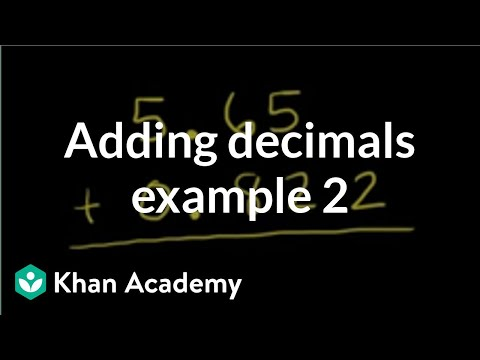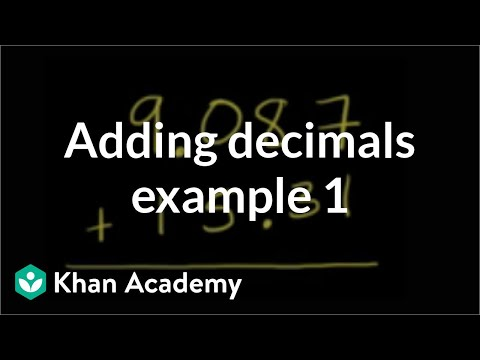Video

# Developing strategies for multiplying decimals (Full video)

Description: Sal uses place value and equivalent fractions to multiply decimals. So right over here we wanna compute what three times 0.25 or three times 25 hundredths is. In the future, we're going to show you what's called the standard strategy, which you might use a lot, but the strategies we're gonna look at in this video are actually very helpful for understanding what multiplying decimals actually means, how it relates to multiplying fractions, as often the way that people, even people who have a lot of math behind them, how they actually multiply decimals. One way is to say, hey, this is the same thing as three times, and I'm just gonna write it a different way.

### Other videos you might be interested in### Adding decimals: 0.822 + 5.65 (Full video)